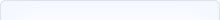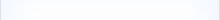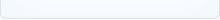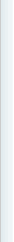The Continuous TensesVerb TensesAn introduction to verbs & personal pronounsVerbs - Ser (to be) Estar (to be)The present simple tense - regular verbs (I am)The present simple tense - irregular verbs (I am)The past simple tense - regular verbs (I was)The past simple tense - irregular verbs (I was)The imperfect tense - all verbs (I used to)The future tense - all verbs (I will be)The continuous tenses (I am going)The perfect tenses - regular and irregular verbsThe conditional tense - regular and irregular verbsThe present subjunctive tenseThe past subjunctive tense (If I were to)The infinitive tense (verbs in their original forms - to be)The imperative tense (command verbs - go / stay etc)'Haber' with the conditional and past subjunctive tenses More About VerbsReflexive verbs - Part 1Reflexive verbs - Part 2The 'Gerund' (the equivalent of forming 'ing...' verbs)'Gustar' and similar verbs - A different way of using verbs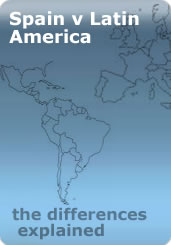All About ArticlesArticles - definite / indefinite ('the' and 'a' in English) All About NounsNouns - Part 1 - (Masculine or feminine?)Nouns - Part 2 - (Gender and forming plural nouns) All About AdjectivesAdjectives - (Agreement and word order)Adjectives - (Comparative and superlative) All About PronounsPronouns - object pronouns (direct / indirect) Adjectives & PronounsAdjectives and Pronouns (demonstrative)Adjectives and Pronouns (possessive)Adjectives and pronouns (indefinite) All About AdverbsAdverbs - Part 1 - (words ending in -ly in English)Adverbs - Part 2 - (Other forms / making comparisons) PrepositionsAn introduction to prepositions and relative pronounsPrepositions - A comprehensive list with examples ConjunctionsConjunctions - linking words Questions & NegativesQuestions and negatives (question words)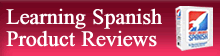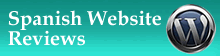Which language learning method best suits you?
 self study classroom learning cultural immersion interactive software a mixture of allSummary of Lesson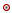A general look at how continuous tenses are formed; (estar+verb+gerund). How the gerund is formed with AR, ER and IR verbs.A look at how ‘estar’ is formed when used with each of the continuous tenses.When continuous tenses are used in conversation and a special look at the present continuous tense. Comparisons with how they are used in English.The continuous tenses as used with ‘haber’. Forming more complex sentences. What you can learn from this lessonUnderstanding how continuous tenses are formed. Being aware of stem changes in irregular verbs and knowing how to correctly form verb endings.Being able to formulate sentences in all continuous tenses by correctly using each form of the verb ‘estar’.Understanding when continuous tenses are used in conversation and when other tenses may be used instead. Knowing how continuous tenses are used in a common way regardless of what other tense they are being used with!Knowing how to correctly position the verb ‘haber’ when used in continuous tense verb constructions. Being able to formulate more complex sentence structures using ‘haber’, with confidence.Affiliates ROCKET SPANISHROSETTA STONE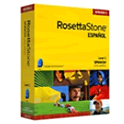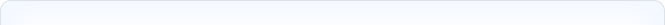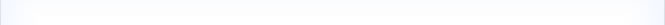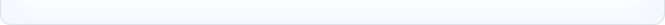PART1

The first part of this lesson looks at what the ‘gerund’ is and how it is used in both Spanish and English to form continuous tenses.

The ‘gerund’ in Spanish is the term given to a verb form whose ending either ends in ‘iendo’ or ‘ando’. (Some irregular ER and IR verbs end in yendo or endo.) In English the equivalent are verbs that end in ‘ing’. Much more information about how ‘gerunds’ are used and formed with verbs is given in a separate lesson.

To form continuous tenses the ‘gerund’ form of a verb is usually used with the verb ‘estar’ (to be). Here are some examples.

 Estoy preguntando I'm asking Estuvimos escogiendo We were choosing Estarán viniendo They will be coming

The formation of the ‘gerund’ depends on whether a verb is regular or irregular. Many verbs that are formed with the ‘gerund’ are regular. See the separate lesson on the ‘gerund’ to see which common verbs are irregular. To illustrate how the ‘gerund’ is used to form continuous tenses, in this lesson we will look at only regular verbs.

Regular verbs are formed as follows:

AR verbs - take off the AR ending and add - ando

caminar (to walk) camin = caminando

terminar (to finish) termin = terminando

ER verbs - take off the ER ending and add - iendo

IR verbs - take off the IR ending and add - iendo

comer (to eat) com = comiendo

aprender (to learn) aprend = aprendiendo

salir (to leave) sal = saliendo

escupir (to spit) escup = escupiendo

To form a continuous tense with ‘gerunds’ the verb ‘estar’ needs to be placed directly in front of the verb + gerund.

 CONTINUOUS TENSE = ESTAR + GERUND VERB FORMATION

The verb + gerund part of the continuous tense never changes. It is the verb ‘estar’ that changes. The way ‘estar’ is used ultimately dictates which continuous tense is being formed. The following illustrates how the verb estar is used with the verb caminar (to walk) to formulate the present continuous tense.

(Yo) estoy caminando = present continuous tense

(I am walking)

PART 2

Part 2 of the lesson looks at how the verb ‘estar’ can be used to form different types of continuous tenses. Different continuous tenses can be formed by simply changing the tense of the verb ‘estar’. By this logic you can see that in reality it would be possible to form many different forms of continuous tenses. The following list shows some of the various possibilities that exist. In each example the verb caminar (to walk), has been used with the personal pronoun yo (I).

 (Yo) estoy caminando. present simple continuous tense (I am walking.) (Yo) estuve caminando. past simple continuous tense (I was walking.) Yo estaba caminando. imperfect continuous tense (I was walking.) (Yo) estaré caminando. future continuous tense (I will be walking.) (Yo) he estado caminando. present perfect continuous tense (I have been walking.) Yo había estado caminando. past perfect continuous tense (I had been walking.) (Yo) habré estado caminando. future perfect continuous tense (I will have been walking.) Él quiere que yo esté caminando. present subjunctive continuous tense. (He wants me to be walking.) Él quiso que yo estuviera caminando. past subjunctive continuous tense. (He wanted me to be walking.) Yo debería estar caminando. conditional continuous tense (should) (I should be walking.) Yo podría estar caminando. conditional continuous tense (could) (I could be walking.) Yo estaría caminando. conditional continuous tense (would). (I would be walking.) (Yo) voy a estar caminando future continuous tense with 'ir' (I’m going to be walking) Yo iba estar caminando past continuous tense with 'ir' (I was going to be walking)

Things to note:

1)      The formation of the verb caminar (to walk), does not change.

2)      The verb estar changes with each tense.

In reality many of the above continuous tenses may seldom be used, or at least not very often in every day speech. It is important to know however, how to formulate continuous tense should the necessity arise.

PART 3

Part three of the lesson looks at when the continuous tenses are commonly used in everyday speech.

Present continuous tense

This tense is used to talk about things that are happening at the very moment at which we are speaking.

Él está viniendo. - He’s coming (right now).

Está quemando. - It’s burning (right now).

Ella está corriendo. - She’s running (right now).

Note: If we want to talk about things which are happening in general or which are not actually happening at this very moment the present simple tense is often used instead of the present continuous tense.

Siempre crecemos. - We are always growing.

(Yo) estudio teatro. - I’m studying drama.

The present continuous tense can also be used to talk about the future in the same way that the present simple tense can.

Estoy trabajando mañana. - I’m working tomorrow.

Están llegando a las siete. - They’re arriving at seven.

Past continuous tense

This tense can often be used instead of using the imperfect tense in Spanish. For more information on the imperfect tense see the separate lesson on the ‘imperfect’ tense. The translation in English in these situations is the same.

Yo caminaba por la calle cuando sucedió.

(Imperfect tense.)

I was walking down the street when it happened.

Yo estaba caminando por la calle cuando sucedió.

(Imperfect continuous tense.)

I was walking down the street when it happened.

In many other situations the formation of continuous tenses are used logically in speech. Often they are formed simply when we want to describe something that is, was, will be, or might be happening over a period of time.

PART 4

The final part of this lesson looks at when the continuous tenses might be used in more complex sentences using ‘haber’ (to have). As always the verb ‘estar’ is used to form the continuous tenses. To understand more about how the verb ‘haber’ is used to form different tenses take a look at the lessons on the ‘perfect’ tenses and ‘haber’ with the conditional and subjunctive tenses.

The important thing to remember here is that the verb ‘haber’ is always placed before the verb ‘estar’.

 (verb) + haber + estar + gerund

There are many ways in which haber can be used to form continuous tenses. Here are a two examples using the verb ‘escuchar’ (to listen).

(You would have been listening.)